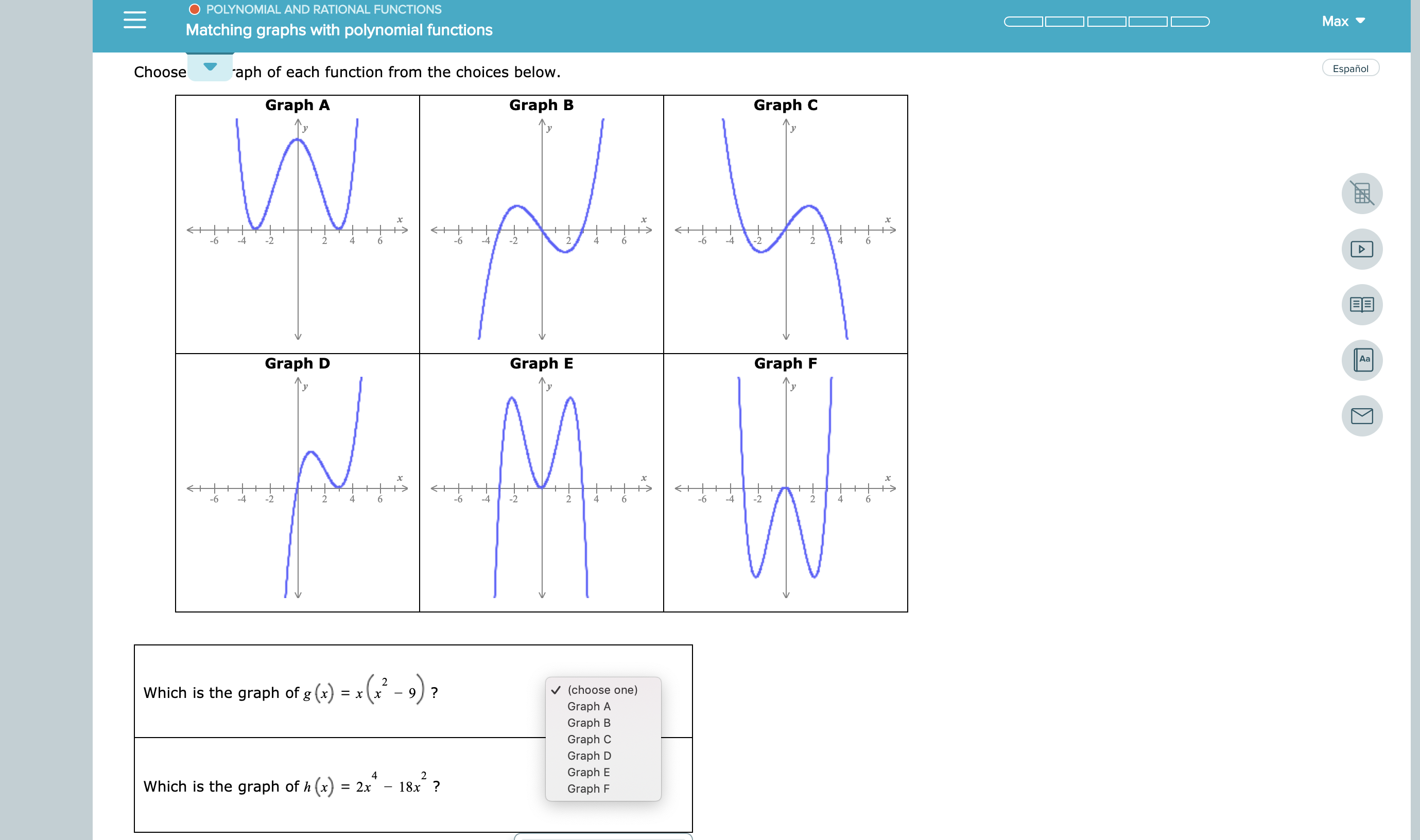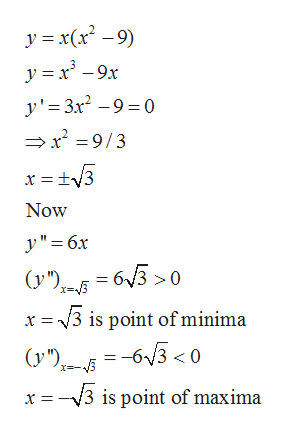# POLYNOMIAL AND RATIONAL FUNCTIONSMaxMatching graphs with polynomial functionsEspañolChooseaph of each function from the choices below.Graph AGraph BGraph C+-6+6+-6-42244-22-224446AaGraph EGraph DGraph F+-6-6-4-2-6-4-244-242Which is the graph of g (x) = x (x-(choose one)Graph AGraph BGraph CGraph DGraph E42Which is the graph of h (x)18x ?2xGraph F

Question
2 views

see attachmenthelp_outlineImage TranscriptionclosePOLYNOMIAL AND RATIONAL FUNCTIONS Max Matching graphs with polynomial functions Español Choose aph of each function from the choices below. Graph A Graph B Graph C + -6 + 6 + -6 -4 2 2 4 4 -2 2 -2 2 4 4 4 6 Aa Graph E Graph D Graph F + -6 -6 -4 -2 -6 -4 -2 4 4 -2 4 2 Which is the graph of g (x) = x (x- (choose one) Graph A Graph B Graph C Graph D Graph E 4 2 Which is the graph of h (x) 18x ? 2x Graph F fullscreen
check_circle

Step 1

We will use the concept of maxima and minima for finding the correct graph. For maxima and minima of curve we first differentiate the curve with respect to x and then find the critical points by equating the derivative zero, after that we find the second derivative of the function and if the second derivative is positive at critical points then we say that the function has minima at that points and if the second derivative is negative at that points then we say that the function has maxima at that points.

Step 2

(a)Given curve ishelp_outlineImage Transcriptionclosey xx-9) y x9x y'3x2-90 x 9/3 Now y"6x ( = 6/3 > 0 x v3 is point of minima -63 <0 (y") (y ") x -3 i point of maxima fullscreen
Step 3

Correct graph of t...

### Want to see the full answer?

See Solution

#### Want to see this answer and more?

Solutions are written by subject experts who are available 24/7. Questions are typically answered within 1 hour.*

See Solution
*Response times may vary by subject and question.
Tagged in

### Calculus